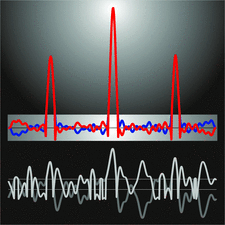issue contentsFOUNDATIONSADVANCES
ISSN: 2053-2733

September 2006 issueCover illustration: Determination of a one-dimensional structure by the charge flipping algorithm. Bottom curves: grey is the initial electron density obtained with a random choice of structure-factor phases; white is the result of charge flipping, i.e. the sign of the density below a threshold is reversed. Top curves: electron density after convergence (blue) and its flipped counterpart (red). Only the three atomic regions correspond to the true structure, small oscillations do not. See Oszlányi & Süto [Acta Cryst. (2004), A60, 134-141; (2005), A61, 147-152].

research papers

Towards a classification of icosahedral viruses in terms of indexed polyhedra

The surface structures of viral capsids are described in terms of polyhedra with icosahedral symmetry and rational indices. It is shown that the Caspar–Klug and the Twarock approaches can be embedded into this framework. The crystallographic scaling transformations are worked out explicitly and are used to derive the icosahedral forms for some viruses, including the Caspar–Klug and Twarock cases.

Application of maximum likelihood to direct methods: the probability density function of the triple-phase sums. XI

A more general probability density function of the triple-phase sums capable of predicting negative values is derived with the maximum-likelihood method. It is also demonstrated that maximization of the origin-free modulus sum function yields the maximum-likelihood estimates of the phases.

The derivation of twin laws in non-merohedric twins. Application to the analysis of hybrid twins

An algorithm for the derivation of twin laws in non-merohedric twins and for the analysis of the hybrid twins is presented. A Fortran program is available.

Three-periodic nets and tilings: edge-transitive binodal structures

28 nets, and their natural tilings, with two kinds of vertex and one kind of edge are described.

A method for hierarchical comparative analysis of crystal structures

A method is proposed for hierarchical enumeration and topological comparative analysis of all crystallochemically significant representations for compounds of any composition and chemical nature. The advantages of the method are shown by the analysis of typical inorganic compounds and a molecular packing.

Contributions to the application of the transferability principle and the multipolar modeling of H atoms: electron-density study of L-histidinium di­hydrogen orthophosphate ortho­phosphoric acid. I

Electron-density determination of L-histidinium dihydrogen orthophosphate orthophosphoric acid from X-ray and neutron diffraction data. The effect of the refinement strategy on the topological properties of ρ(r) in the hydrogen bonds and the use of the transferability principle to obtain accurate thermal parameters are analysed.

Hexagonal and trigonal sphere packings. IV. Trivariant lattice complexes of trigonal space groups

For the 13 trivariant lattice complexes of the trigonal space groups, all types of homogeneous sphere packings, interpenetrating sphere packings and layers are derived. Examples of related crystal structures are given.

On the calculation of the electrostatic potential, electric field and electric field gradient from the aspherical pseudoatom model

Accurate, simple and efficient formulae for calculation of the electrostatic potential, electric field and electric field gradient from the aspherical pseudoatom model of electron density are presented. The expressions are applied to the determination of the nuclear quadrupole moment of the Fe atom in Fe(CO)5.

short communications

Gaussian binomials and the number of sublattices

This short communication discusses the connections between sublattice counting methods and their relation to Gaussian binomials.

Recurrence properties of O-lattices and the classification of grain boundaries

A recurrence relation is shown to exist between O-lattices that induces a partition of the angular space into a finite number of equivalence classes. This establishes a much sought after general classification for rotation-related interfaces derived from first principles.
Follow Acta Cryst. A